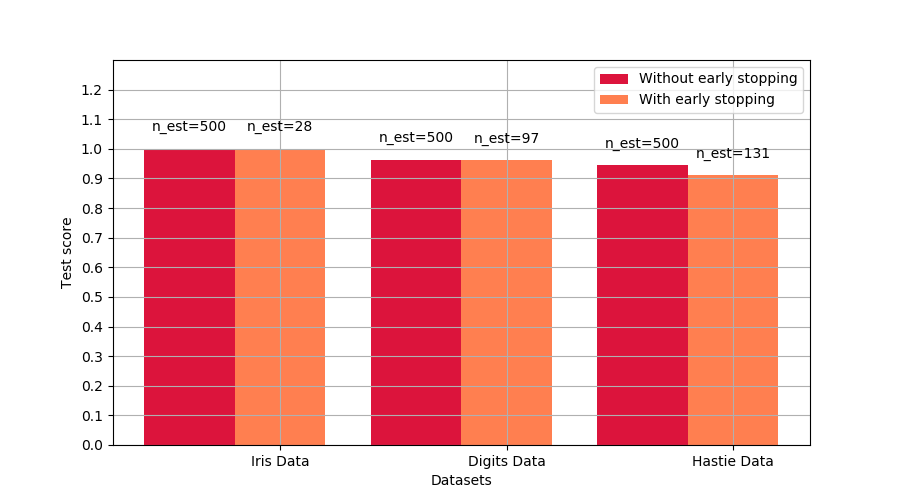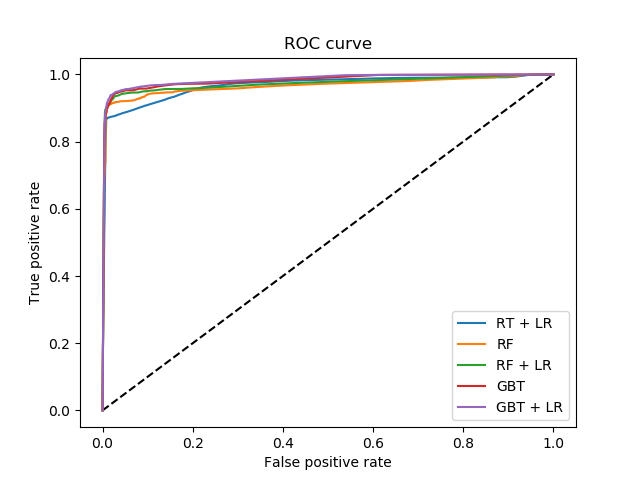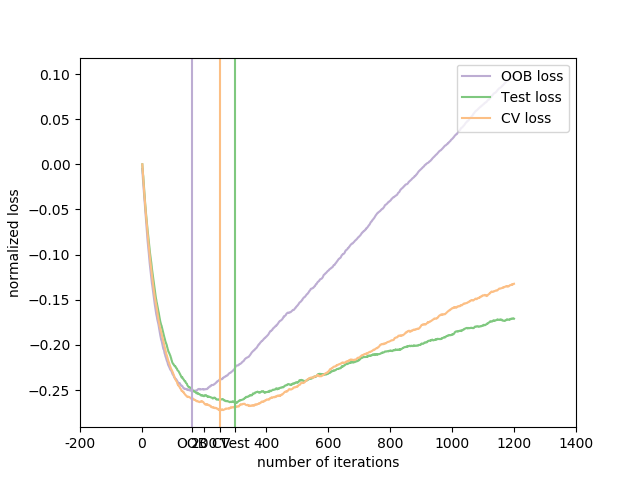class sklearn.ensemble.GradientBoostingClassifier(*, loss='deviance', learning_rate=0.1, n_estimators=100, subsample=1.0, criterion='friedman_mse', min_samples_split=2, min_samples_leaf=1, min_weight_fraction_leaf=0.0, max_depth=3, min_impurity_decrease=0.0, min_impurity_split=None, init=None, random_state=None, max_features=None, verbose=0, max_leaf_nodes=None, warm_start=False, presort='deprecated', validation_fraction=0.1, n_iter_no_change=None, tol=0.0001, ccp_alpha=0.0)

GB采用正向阶段的方式建立加性模型;它允许优化任意可微的损失函数。在每一阶段，n_classes_回归树都拟合在二项或多项偏差损失函数的负梯度上。二元分类是一种特殊的情况，其中只有一个回归树被诱导。

loss {‘deviance’, ‘exponential’}, default=’deviance’

learning_rate float, default=0.1

n_estimators int, default=100

subsample float, default=1.0

criterion {‘friedman_mse’, ‘mse’, ‘mae’}, default=’friedman_mse’

min_samples_leaf 分割一个内部节点所需的最小样本数:
- 如果为int，则认为min_samples_leaf是最小值。
- 如果为float，min_samples_leaf则为分数, 是每个节点的最小样本数。
ceil(min_samples_leaf * n_samples)

min_samples_split int or float, default=1

- 如果为int，则认为min_samples_split是最小值。
- 如果为float，min_samples_split则为分数， 是每个拆分的最小样本数。
ceil(min_samples_split * n_samples)

min_weight_fraction_leaf float, default=0.0

max_depth int, default=3

min_impurity_decrease float, default=0.0

N_t / N * (impurity - N_t_R / N_t * right_impurity - N_t_L / N_t * left_impurity)

NN_tN_t_R并且N_t_L都指的是加权和，如果sample_weight获得通过。

min_impurity_split float, default=None

- 从版本0.19min_impurity_split开始不推荐使用：在版本0.19中不再推荐使用 min_impurity_decrease。的默认值 min_impurity_split在0.23中从1e-7更改为0，并将在0.25中删除。使用min_impurity_decrease代替。
init estimator or ‘zero’, default=None

random_state int, RandomState, default=None

max_features {“auto”, “sqrt”, “log2”}, int or float, default=”auto”

- 如果为int，则max_features在每个分割处考虑特征。
- 如果为float，max_features则为小数，并 在每次拆分时考虑要素。
- 如果为auto，则为max_features=sqrt(n_features)
- 如果是sqrt，则max_features=sqrt(n_features)
- 如果为log2，则为max_features=log2(n_features)
- 如果为None，则max_features=n_features

verbose int, default=0

max_leaf_nodes int, default=None

warm_start bool, default=False

presort deprecated, default=’deprecated’

validation_fraction float, default=0.1

0.20版中的新功能。
n_iter_no_change int, default=None
n_iter_no_change用于决定在验证分数没有提高时是否使用早期停止来终止训练。默认情况下，它被设置为None来禁用提前停止。如果设置为一个数字，它将把训练数据的validation_fraction大小放在一边作为验证，并在之前所有的n_iter_no_change迭代次数中验证分数没有提高时终止训练。分裂是分层的。
0.20版中的新功能。
tol float, default=1e-4

0.20版中的新功能。
ccp_alpha non-negative float, default=0.0

0.22版中的新功能。

n_estimators_ int

0.20版中的新功能。
feature_importances_ ndarray of shape (n_features,)

oob_improvement_ ndarray of shape (n_estimators,)

train_score_ ndarray of shape (n_estimators,)
i个得分train_score_[i]是模型在第i次迭代时对袋装样本的偏差度(= loss)。如果subsample== 1，这便是训练数据上的偏差。
loss_ LossFunction

init_ estimator

estimators_ ndarray of DecisionTreeRegressor of shape (n_estimators, loss_.K)

classes_ ndarray of shape (n_classes,)

n_features_ int

n_classes_ int or list

max_features_ int
max_features的推断值。

sklearn.ensemble.HistGradientBoostingClassifier

AdaBoostClassifier

J. Friedman, Greedy Function Approximation: A Gradient Boosting Machine, The Annals of Statistics, Vol. 29, No. 5, 2001.

​ 10. Friedman, Stochastic Gradient Boosting, 1999

T. Hastie, R. Tibshirani and J. Friedman. Elements of Statistical Learning Ed. 2, Springer, 2009.

>>> from sklearn.datasets import make_classification>>> from sklearn.ensemble import GradientBoostingClassifier>>> from sklearn.model_selection import train_test_split>>> X, y = make_classification(random_state=0)>>> X_train, X_test, y_train, y_test = train_test_split(...     X, y, random_state=0)>>> clf = GradientBoostingClassifier(random_state=0)>>> clf.fit(X_train, y_train)GradientBoostingClassifier(random_state=0)>>> clf.predict(X_test[:2])array([1, 0])>>> clf.score(X_test, y_test)0.88

apply(X) 将森林中的树应用于X，返回叶子索引。
decision_function(X) 计算X的决策函数。
fit(X, y[, sample_weight, monitor]) 拟合gradient boosting模型。
get_params([deep]) 获取此估计器的参数。
predict(X) 预测X的类。
predict_log_proba(X) 预测X的类对数概率。
predict_proba(X) 预测X的类概率。
score(X, y[, sample_weight]) 返回给定测试数据和标签上的平均准确度。
set_params(**params) 设置此估算器的参数。
staged_decision_function(X) 每次迭代计算X的决策函数。
staged_predict(X) 预测X在每个阶段的分类。
staged_predict_proba(X) 预测X在每个阶段的类概率。
__init__(*, loss='deviance', learning_rate=0.1, n_estimators=100, subsample=1.0, criterion='friedman_mse', min_samples_split=2, min_samples_leaf=1, min_weight_fraction_leaf=0.0, max_depth=3, min_impurity_decrease=0.0, min_impurity_split=None, init=None, random_state=None, max_features=None, verbose=0, max_leaf_nodes=None, warm_start=False, presort='deprecated', validation_fraction=0.1, n_iter_no_change=None, tol=0.0001, ccp_alpha=0.0)

[源码]

apply(X)

[源码]

0.17版本的新功能

X {array-like, sparse matrix} of shape (n_samples, n_features)

X_leaves array-like of shape (n_samples, n_estimators, n_classes)

decision_function(X)

[源码]

X {array-like, sparse matrix} of shape (n_samples, n_features)

score ndarray of shape (n_samples, n_classes) or (n_samples,)

property feature_importances_

feature_importances_ ndarray of shape (n_features,)

fit(X, y, sample_weight=None, monitor=None)

[源码]

X {array-like, sparse matrix} of shape (n_samples, n_features)

y array-like of shape (n_samples, )

sample_weight array-like of shape (n_samples,), default=None

monitor callable, default=None

self object
get_params(deep=True)

[源码]

deep bool, default=True

params mapping of string to any

predict(X)

[源码]

X {array-like, sparse matrix} of shape (n_samples, n_features)

y ndarray of shape (n_sample, )

predict_log_proba(X)

[源码]

X {array-like, sparse matrix} of shape (n_samples, n_features)

p ndarray of shape (n_samples, n_classes)

Raises AttributeError
loss不支持概率。
predict_proba(X)

[源码]

X {array-like, sparse matrix} of shape (n_samples, n_features)

p ndarray of shape (n_samples, n_classes)

Raises AttributeError
loss不支持概率。
p ndarray of shape (n_samples, n_classes)

score(self, X, y, sample_weight=None)

[源码]

X array-like of shape (n_sample, n_features)

y array-like of shpe (n_sample, ) or (n_samples, n_outputs)
X的正确标签。
sample_weight array-like of shape (n_samples, ), default = None

score float
self.predict(X) 关于y的平均准确率。
set_params(**params)

[源码]

**params dict

self object

staged_decision_function(X)

X {array-like, sparse matrix} of shape (n_samples, n_features)

score generator of ndarray of shape (n_samples, k)

staged_predict(X)

[源码]

X {array-like, sparse matrix} of shape (n_samples, n_features)

y generator of ndarray of shape (n_samples, )

staged_predict_proba(self, X)

[源码]

X {array-like, sparse matrix} of shape (n_samples, n_features)

p generator of ndarray of shape (n_samples,)